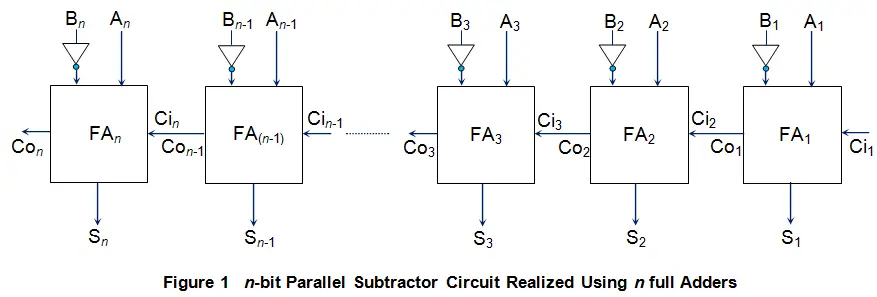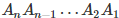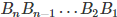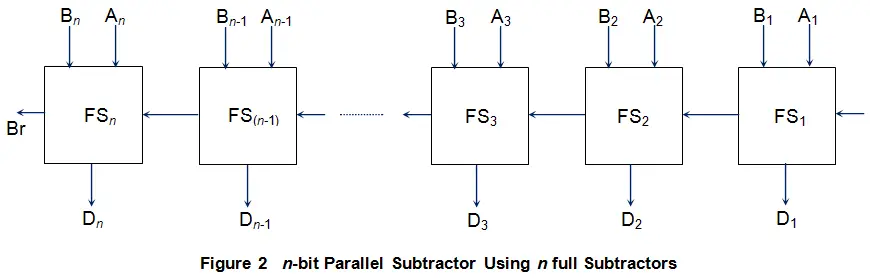# Parallel Subtractor

The combinatorial circuits which are used to subtract two binary numbers are called Subtractors. When the binary numbers to be subtracted are of single bits, then, we can use a half subtractor to accomplish the task while if we need to subtract three binary numbers of single bits (among which two will generally be inputs while the other will be the borrow), we will have to use full subtractor. Now what if we desire to subtract two n-bit binary numbers? This is the case which demands for the use of n-bit parallel subtractor.

## Structure of Parallel Subtractor

Generally when one needs to subtract the binary number 2 from binary number 1, then the binary number 2 will be expressed in its 2’s complement form and then added with the binary number 1 (nothing but 2’s complement form of binary subtraction).

Next, 2’s complement of a number can be obtained by taking 1’s complement of the number and then by adding 1 to its least significant bit (LSB). Further, taking 1’s complement means nothing but negating the binary number. From the discussion presented, one can conclude that inorder to accomplish subtraction, one can use the same circuit as that for addition (more on this in the article “Parallel Adder”) provided we have the number ‘to be subtracted’ in its 2’s complement form. This task of expressing the number in 2’s complement form may be brought about by first using NOT gates to invert the bits in the binary number. However, to add 1 at LSB, one can make use of the first adder in the sequence of n full adders used in the circuit just by providing logic high at its carry in (Ci1) pin. As a result, one can design the n-bit parallel subtractor as shown in Figure 1.Here the binary numberis the minuend and the binary numberis the subtrahend. Further, the sum outputs of each and every adder actually correspond to the difference bits (the expected result) while the carry out pin of the last full adder (Con) will be nothing but the resultant borrow.

Apart from this kind of circuit, one can even design the parallel subtractor using just a cascaded array of full subtractors. Figure 2 shows such an-bit parallel subtractor designed using n full subtractors (FS1 to FSn) joined in a way similar to that of in the case of n-bit parallel adder.The working of such a circuit is straight forward and is very similar to that of a parallel adder. As a result, even parallel subtractors are prone to the effect of ripple propagation which results in the delayed output. That is, if the dealy associated with each of the full subtractor is T seconds, then the overall difference bits (D1D2…Dn) and borrow bit (Br) are obtained only after n T seconds.

Want To Learn Faster? 🎓
Get electrical articles delivered to your inbox every week.
No credit card required—it’s 100% free.